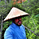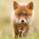1154 뷰
1154
EXPERIMENTAL:
Double EMA RSI Strategy.
Optional:
N max orders per day, %equity trade size, Time session constraints, TP, SL, Trailing.
```//@version=2
strategy(title='[STRATEGY][RS]DemaRSI V0', shorttitle='D', overlay=false, initial_capital=100000, currency=currency.USD)
src = input(close)
ma_length = input(21)
rsi_length = input(4)
rsi_smooth = input(4)

ma = ema(ema(src, ma_length), ma_length)
marsi = rsi(ma, rsi_length)
smooth = ema(marsi, rsi_smooth)
plot(title='M', series=marsi, color=black)
plot(title='S', series=smooth, color=red)
hline(0)
hline(50)
hline(100)

max_order_per_day = input(6)
take_profit_in_points = input(100000)
stop_loss_in_points = input(100000)
trail_in_points = input(150)

USE_SESSION = input(true)

sel_entry = istradingsession and crossunder(marsi, smooth)

strategy.exit('sel.Exit', from_entry='sel', profit=take_profit_in_points, loss=stop_loss_in_points, trail_points=trail_in_points, trail_offset=trail_in_points)How does 'trail_offset=trail_in_points' work? Cant get the darn thing to work for some reason
응답ArgosyExponential
its the value that price has to track back in points(1/10 of a pip), trail is on by default unless you put a lower value on the stop loss.
응답RicardoSantos
misread, offset is the distance from open to the positive to activate trailling stop.
응답RicardoSantos
any ability to set it in % terms rather than ticks/pips?
응답